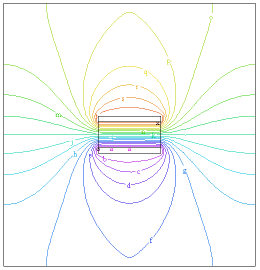﻿ Sample Problems > Applications > Electricity > plate_capacitor

# plate_capacitor

Navigation:  Sample Problems > Applications > Electricity >

# plate_capacitor{  PLATE_CAPACITOR.PDE

This problem computes the field around a plate capacitor.

(adapted from "Fields of Physics on the PC" by Gunnar Backstrom)

}

 title 'Plate capacitor' variables  u definitions  Lx=1      Ly=1  delx=0.5  d=0.2  ddy=0.2*d  Ex=-dx(u)     Ey=-dy(u)  Eabs=sqrt(Ex^2+Ey^2)  eps0=8.854e-12  eps  DEx=eps*Ex    DEy=eps*Ey  Dabs=sqrt(DEx^2+DEy^2)  zero=1.e-15 equations  u : div(-eps*grad(u)) = 0 boundaries Region 1    eps=eps0   start "V0" (-Lx,-Ly)       Load(u)=0line to (Lx,-Ly) to (Lx,Ly) to (-LX,Ly) to close

start "V1" (-delx/2,-d/2)

value(u)=0

line to (delx/2,-d/2) to (delx/2,-d/2-ddy) to (-delx/2,-d/2-ddy)

to close

start(-delx/2,d/2+ddy)

value(u)=1

line to (delx/2,d/2+ddy) to (delx/2,d/2) to(-delx/2,d/2)

to close

Region 2

eps = 7.0*eps0

start(-delx/2,-d/2)

line to (delx/2,-d/2) to (delx/2,d/2) to(-delx/2,d/2)

to close

monitors

contour(u)

plots

contour(u)

surface(u)

vector(dx(u),dy(u))

fieldmap(u) on "V1" fieldlines=40 as "Field Map"

contour fieldmap(u) on "V1" fieldlines=40 points=400 as "Potential and Field Map"

end